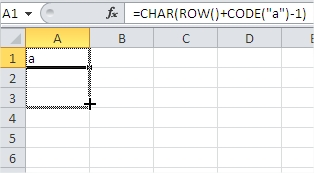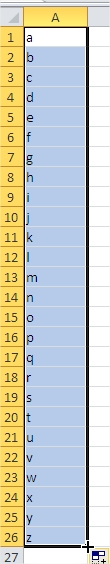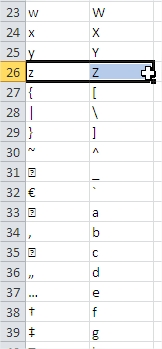#### How to Insert Alphabet in Excel

In Excel, in a very simple way you can generate the entire alphabet.

## The formula

=CHAR(ROW()+CODE(“A”)-1)

=CHAR(ROW()+CODE(“a”)-1)

If you want to generate the alphabet in capital letters, type “A”.

If you want to generate a lowercase alphabet, type “a”.

Enter one of the following formulas into a cell and drag the cell via drag & drop.Excel will automatically fill in the remaining letters of the alphabet for you.## Examples

If you drag more than 26 cell, Excel will insert only the letter.

If you drag exactly 26 cell, Excel will insert through the entire alphabet.

If you drag more than 26 cell, Excel will insert other trademarks charset. These signs are different for upper- and lowercase letters.This trick could be useful for developers and analysts who are dealing with alphabet or charset in spreadsheets.

## CHAR and ROW formula

Alternatively, you can use a formula to insert the alphabet. For example, the following formula will generate the alphabet from A to Z in separate cells:

=CHAR(ROW(A1)+64)

Copy and paste this formula into the first cell and then use the AutoFill feature to fill it down to the last cell where you want the alphabet to be inserted.

Note that you can use the CHAR function to insert other characters as well, such as special symbols or punctuation marks, by using the appropriate ASCII code. The ASCII code for A is 65, so the formula above subtracts 64 to get the ASCII code for A.